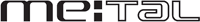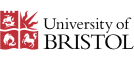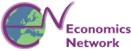# Film Series Two: Linear Equations

The economist's perspective in understanding the decisions of consumers is that people bring their preferences to the marketplace and make purchases that will maximise their sense of satisfaction, or utility. However, our choices are constrained by a limited income and by the price we have to pay to make any purchase. This series of films uses linear equations to explore constraints in markets.

These video clips and animations can be viewed in isolation, or you can use our learning pathway and follow links to related material in MathsEG.

 1 Watch or downloadthe video & animation 2  View related question material

#### 2.01 Linear Functions - Budget Lines

The use of linear functions to examine the spending options for a university student. (11 minutes 30 seconds).

#### 2.02 Linear Consumption Functions - East and West Germany

Average income between regions in Germany varies greatly. The effects of these differences on expenditure patterns are examined by linear consumption functions. (9 minutes and 5 seconds).

#### 2.03 Linear Savings Functions - East and West Germany

Savings patterns in East and West Germany are related to income using a linear savings function. (5 minutes and 12 seconds).

#### 2.04 Summary on Linear Functions

A summary of the significance of linear functions in economics. (54 seconds).

#### 2.05 Linear Equations in Goods and Labour Markets

A review of linear equations in order to find equilibrium quantities. (7 minutes 26 seconds).

#### 2.06 Solving Simultaneous Equations - French Wine

The price of French wine can be explained using supply and demand using simultaneous equations. (7 minutes 6 seconds).

#### 2.07 Linear Equations - Taxes and Subsidies in Wine Markets

The effects on price and output of government subsidies in French and American wine production. (8 minutes 3 seconds).

 View, rate or comment on YouTube Permalink Download (60 MB) Flash animation only Related MathsEG material Linear Simultaneous Equations Supply and Demand/ Linear relations

#### 2.08 Summary - Solving Linear Equations

Summary - Solving linear equations. (33 seconds).

#### 2.09 Solving Simultaneous Equations - Labour Markets

Using simultaneous equations to understand the wage rate for workers on ferries between Dover and Calais

#### 2.10 Solving Simultaneous Equations - Trade Unions and Minimum Wages

Using simultaneous equations to understand the effects of trade unions and minimum wage laws on wage rates.

#### 2.11 Simultaneous Equations - Summary

A summary of the use of two simultaneous equations.

#### 2.12 Simultaneous Equations - More Than Two

The decision to purchase a student rail card provides an illustration of the use of more than two linear equations. (9 minutes 29 seconds).

 View, rate or comment on YouTube Permalink Download (58 MB) Flash animation only Related MathsEG material Linear Simultaneous Equations Supply and Demand/ Linear relations

#### 2.13 Simultaneous Equations - Production and Cost in Swedish Truck Production

Profitability in Swedish forklift truck manufacture is explained using more than two simultaneous equations. (9 minutes and 27 seconds).

 View, rate or comment on YouTube Permalink Download (72 MB) Flash animation only Related MathsEG material Linear Simultaneous Equations Supply and Demand/ Linear relations

#### 2.14 Simultaneous Equations - Production and Cost in Bulgarian Truck Production

Break-even points in Bulgarian forklift truck manufacture are explained using more than two simultaneous equations. (5 minutes and 56 seconds).

 View, rate or comment on YouTube Permalink Download (40 MB) Flash animation only Related MathsEG material Linear Simultaneous Equations Supply and Demand/ Linear relations

#### 2.15 Conclusion

A summary of how simultaneous equations are used in elementary economics. (2 minutes 54 seconds).